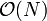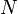# Difference between revisions of "Queue"

Jump to: navigation, search

A queue is a data structure containing zero or more items, all of the same type, which supports two basic operations:

• Push an element onto the queue.
• Pop the first value off the queue, returning its value in the process.

In practice, we might choose to include the following operations as well:

• Construct an empty queue.
• Copy the contents of one queue to another.
• Peek at the first element in the queue (the one which would be popped next), without removing it.
• Empty an already existing queue.
• Find size of a queue.
• Test if empty.

Unlike with a stack, a peek operation cannot be implemented on a queue as a pop followed by a push. (The test if empty operation is still the same as the find size operation followed by a test of whether or not the size is zero.)

## Terminology

The term queue is based off of real-life queues; they are often referred to as lines, as in the case of a queue for a ride in an amusement park. In a queue like this, we join the end of the queue (become last) and wait until we are at the front (become first) before we are popped (let in). In computer science, the most recently pushed element is last, whereas the least recently pushed element is first. An element can be described as before another if the latter was pushed later; the latter is referred to as being after the former. To push means to add an element, and to pop means to remove an element.

## Implementation

### Array implementation

In an array implementation of a queue, the contents of the queue are stored in consecutive indices in an array. However, we encounter a problem if we want the element in the lowest-indexed position (i.e. 0 or 1) to be constantly at the front: when we pop, we have to shift over all the other elements so that the lowest-indexed position contains the next element to be popped and all elements remain contiguous. This can take$\mathcal{O}(N)$ time where$N$ is the number of elements currently in the queue. But we need constant time push/pop operations in order to make important algorithms like BFS run in linear time. To solve this, we do not replace the popped element, we merely increment an index into the next element to be popped. That is, we maintain two indices into the array: one to the front and one to the back. When we push, we add an element to the back and increment the back index; when we pop, we remove the element from the front and increment the front index. However, consider what happens if, for example, we continually push one element, then pop one, then push another, and pop another, and so on: the size of the queue doesn't change much, but both indices will eventually go out of range. To fix this problem, we allow the indices to wrap around, so that incrementing the highest possible index will give the lowest possible one. This is done using the modulo operation. Following is an exemplary implementation:

object queue
function construct(max_size)
let A be an array that can hold at least max_size+1 elements
let N = max_size+1
let first = 0
let last = 0
function push(x)
A[last] = x
last = (last + 1) mod N
function pop
return_val = A[first]
first = (first + 1) mod N
return return_val
function peek
return A[first]
function size
return (last-first) mod N
function make_empty
last = first


Notice that if the queue becomes full and we attempt to push more elements, then the apparent size will wrap to zero and the earliest-pushed elements will be overwritten. Also, if we attempt to pop an empty queue, the apparent size will wrap to the largest possible value. Error-checking code is fairly simple to add but, like the test if empty and copy operations, it has been omitted for the sake of brevity.

### Linked list implementation

The circular array implementation will almost always be appropriate. However, for rare instances in which a contiguous block of memory of the required size cannot be found, a linked list can be used instead. Details of the list implementation are omitted below:

object queue
function construct
let L be an empty linked list
function push(x)
insert x after tail of L
function pop
remove head of L, returning its data element
function peek
return data element at head of L
function size
return size of L
function make_empty
empty L


As one can easily see, the queue is merely a container over the operations supported by the list itself. (Here we assume that the list maintains its own size; if this is not so then we can add a size field to the queue object.)

### C++ STL queue class

In the C++ STL, std::queue, found in the <queue> header, is a template container capable of holding items of any (fixed) type, the type being supplied as a template argument. Hence, for example, queue<char> is a queue of characters. The container supplies the following member functions (note that T is the type of the elements of the queue):

• void push(const T& x): pushes x into the queue.
• void pop(): performs a pop.
• T& front(): returns the element which is to be popped next
• T& back(): returns the element which was last pushed
• size_type size(): returns the number of elements currently in the queue
• bool empty(): returns true if the queue is empty, false otherwise.

The queue container should be used whenever it is sufficient for the problem at hand; it is quicker and less error-prone to use it than to code your own queue. There are, however, times when it might be necessary to execute some unsupported operation such as iteration through the elements of the queue. In such circumstances the STL queue cannot be used and a custom queue must be implemented.

Note that the pop operation defined by this container is not identical to our own; it only removes the element and it does not return its value. To do both, call front followed by pop.